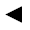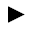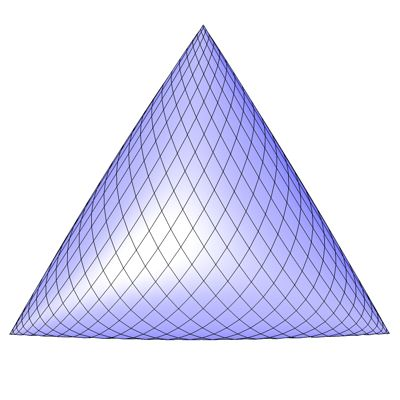﻿ math handbook calculator - Fractional Calculus Computer Algebra System software+++=[back]

## 3.35. Cosine Surface

[next]The cosine surface [ 11 ] is represented by the following equations.

 x = cos(u) 3-120 y = cos(v) 3-121 z = cos(u + v) 3-122

The cosine surface is the counterpart to the sine surface .

To represent the area, the two parameters u and v can assume the following values ​​(domain of definition), for example.

 u is an element from the number set [-pi, pi] v is an element from the number set [-pi, pi]

Since the cosine surface is a closed figure, the definition range must be adhered to exactly, it cannot be changed with the plugin.Fig. 39